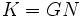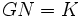# Sylow's theorem with operators

This article states and (possibly) proves a fact that involves two finite groups of relatively prime order, requiring the additional datum that at least one of them is solvable. Due to the Feit-Thompson theorem, we know that for two finite groups of relatively prime orders, one of them is solvable. Hence, the additional datum of solvability can be dropped. However, the proof of the Feit-Thompson theorem is considered heavy machinery.
View more such facts
This article states and (possibly) proves a fact about a finite group and a Coprime automorphism group (?): a subgroup of the automorphism group whose order is relatively prime to the order of the group itself.
View other such facts

## Statement

Suppose$G$ is a finite group and$H$ is a coprime automorphism group of$G$:$H$ is a subgroup of$\operatorname{Aut}(G)$ such that the orders of$G$ and$H$ are relatively prime. A subgroup of$G$ is termed$H$-invariant if it equals its image under any element of$H$.

Suppose further that either$G$ or$H$ is solvable.

Let$p$ be any prime.

1. Existence (E): The set of$H$-invariant$p$-Sylow subgroups of$G$ is nonempty.
2. Conjugacy (C): Any two$H$-invariant$p$-Sylow subgroups of$G$ are conjugate by an element in$C_G(H)$.
3. Domination (D): Any$H$-invariant$p$-subgroup of$G$ is contained in a$H$-invariant$p$-Sylow subgroup of$G$.

Note that since given two groups of coprime order, one of them is solvable, the assumption that either$G$ or$H$ is solvable is redundant.

## Facts used

1. Sylow subgroups exist
2. Frattini's argument
3. Normal Hall implies permutably complemented
4. Hall retract implies order-conjugate: This states that any two complements to a normal Hall subgroup are conjugate. The proof of this is what requires the assumption that either the normal Hall subgroup or the quotient group is solvable.
5. Normal Hall satisfies transfer condition

## Proof

### Proof of existence

Given: A finite group$G$, a subgroup$H$ of$\operatorname{Aut}(G)$ such that$G$ and$H$ have relatively prime orders. A prime$p$.

To prove: There exists a$H$-invariant$p$-Sylow subgroup of$G$.

Proof: Let$K$ be the semidirect product of$G$ with$H$.$G$ is a normal Hall subgroup of$K$, and$G/K \cong H$.

1. By fact (1),$G$ has a$p$-Sylow subgroup, say$P$.
2. Let$N = N_K(P)$. Then,$K = GN$: This follows from fact (2), and the fact that$G$ is normal in$K$.
3. There exists a permutable complement$L$ to$G \cap N$ in$N$: Since$G$ is normal Hall in$K$,$G \cap N$ is normal Hall in$N$. Fact (3) thus applies.
4.$L$ is a permutable complement to$G$ in$K$: Since$GN = K$, we get$G((G \cap N)L) = K$. Since$G \cap N \le G$, this yields$GL = K$. Further,$L \le N$, so$G \cap L \ge G \cap L \cap N = (G \cap N) \cap L$, which is trivial from the previous step.
5.$L$ is conjugate to$H$ by an element of$K$. Since$GH =K$,$L$ and$H$ are conjugate via an element of$G$: This follows from fact (4), and the previous step, which shows that$L$ is also a permutable complement to$G$ in$K$.
6. Let$g$ be the element of$G$ conjugating$L$ to$H$. Let$Q$ be the conjugate of$P$ by$g$. Then,$N_K(Q) = H$: This follows from the fact that conjugation, being an automorphism, preserves normalizers.
7.$Q$ is a$H$-invariant$p$-Sylow subgroup of$G$: This is an immediate corollary of the preceding step.